SYLLABUS  Previous: 4.4 Methods for European  Up: 4.4 Methods for European  Next: 4.4.2 Improved scheme using

4.4.1 Naive implementation using financial variables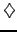[ SLIDE Derivatives 1st - 2nd || Black-Scholes scheme - code - run || VIDEO modem - LAN - DSL]

Start with a finite number prices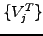that are all known from the terminal condition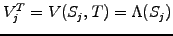with a regular sampling of the underlying asset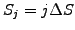. The idea behind the finite difference method is to approximate the infinitesimal changes in the Black-Scholes equation by small (but finite) differences and to generate new prices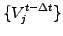in a sequence of small steps taken backward in time until the solution is found. It is not difficult to propose approximations of the partial derivatives directly from the definition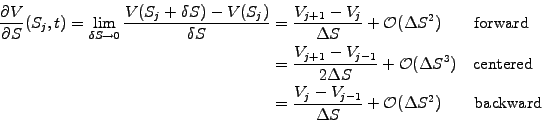(4.4.1#eq.1)

Convince yourself that if you subtract two Taylor expansions around the asset price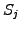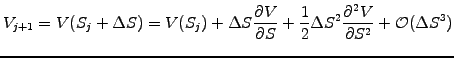(4.4.1#eq.2)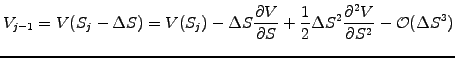(4.4.1#eq.3)

the quadratic terms cancel out, showing that a higher precision in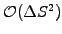is achieved when using a centered scheme. Precision is however not the only requirement and it turns out that only backward (alt. forward) differences are stable for the approximation of the time derivative when the scheme runs backward (alt. forward). An approximation for the second derivative is obtained by subtracting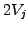from the sum of two Taylor expansions above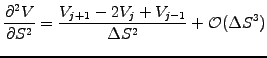(4.4.1#eq.4)

Substituting finite differences for all the partial derivatives in the Black-Scholes equation without dividend (extension considered in exercise 4.07)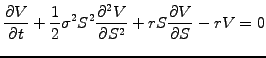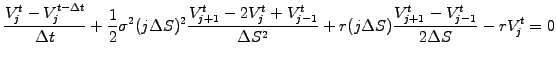(4.4.1#eq.5)

where the time derivative (first term) has been approximated with a difference backward from the time level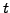for stability reasons and the price derivative (third term) has been centered onfor a higher precision. After a few rearrangements, the unknown new'' option value at a time level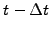are obtained explicitly in terms of the known old'' values at time level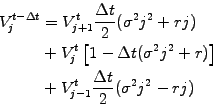(4.4.1#eq.6)

The value of an option can therefore be approximated before the expiry date, using a sequence of small steps to evolve the solution backward until the chosen time is reached.
To complete the formulation, the solution has to be specified on the domain boundaries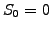and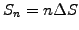; in the same way as for the terminal conditions, these boundary conditions depend on the type of contract. For example, the underlying price at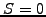doesn't change in the case of log-normal price increments, showing that the value of a put option is certain to be exercised with a payoff equal to the strike price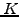. This can be discounted back from the expiry time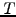at a rate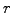. On the contrary, the put option is increasingly unlikely to be exercised if the underlying asset value moves above the strike price and looses all of its value when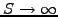. The boundary conditions for a put option without dividends (extension in exercise 4.07) are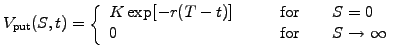(4.4.1#eq.7)

A similar reasoning leads to the boundary conditions for a call option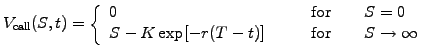(4.4.1#eq.8)

This scheme that has been implemented in the VMARKET class FDSolution.java as
double timeStep = runData.getParamValue("TimeStep");
double strike   = runData.getParamValue("StrikePrice");
double rate     = runData.getParamValue("SpotRate");
double divid    = runData.getParamValue("Dividend");
double sigma    = runData.getParamValue("Volatility");
double sigmaSq  = sigma*sigma;
for (int j=1; j<n; j++) {                          //Explicit 2 levels
fp[j]=f[j+1]* 0.5*timeStep*(sigmaSq*j*j + rate*j)
+f[j  ]*(1.0-timeStep*(sigmaSq*j*j + rate  ))
+f[j-1]* 0.5*timeStep*(sigmaSq*j*j - rate*j);
}                                                  //Boundary condition
if (isCall)      { fp=0;  fp[n]=n*dx -strike*Math.exp(-rate*time);
} else if(isPut) { fp=strike*Math.exp(-rate*time);   fp[n]=fp[n-1];
} else           { fp=fp;  fp[n]=fp[n-1]; }
showing clearly how the option values at the new time level fp[j] are explicitly calculated in terms of the old values f[j]. To limit the required size of the simulation domain around the strike price, it is marginally better to replace the Dirichlet condition fp[n]=0 with a Neumann condition fp[n]=fp[n-1]. This is what has been used above for the put option and in the default, so as to accommodate in a simple manner for more general payoffs from binary options. The VMARKET applet below shows an application using this very simple model.

 Virtual market experiments: naive FD scheme Switch from Put to Call, SuperShr and VSpread to verify that the same scheme works also for binary options. Imagine how the solutions would look like if Dirichlet conditions were used instead of Neumann conditions; note that neither converges to the correct value at the boundaries where the mesh is truncated. Switch back to Put option and try to accelerate the calculation with a slightly larger TimeStep=5E-4. Check how an instability grows for the largest values of the underlying and becomes visible after Time=0.22. Increase the time step above TimeStep=2.74E-3 and switch between different initial conditions to verify how, for large steps, the instability always appears around the largest value where the initial condition is not zero.

The mere simplicity of this naive formulation makes this explicit finite difference scheme attractive; two major problems, however, should lead you to reconsider an early judgment. First, the regular spacing of the grid is ill suited to accommodate a log-normal distribution of price increments: the relative numerical accuracy in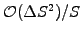rapidly decreases and becomes insufficient when the value of the underlying becomes small. The second problem is the numerical instability, which appears when the solution evolves too rapidly. It turns out that the upper limit on the time step depends on the relative changes that are possible for the largest values of the underlying-even if the option price is negligible there. These drawbacks motivate a transformation to log-normal variables in the same manner as for the analytical solution in section 4.3.1.

SYLLABUS  Previous: 4.4 Methods for European  Up: 4.4 Methods for European  Next: 4.4.2 Improved scheme using